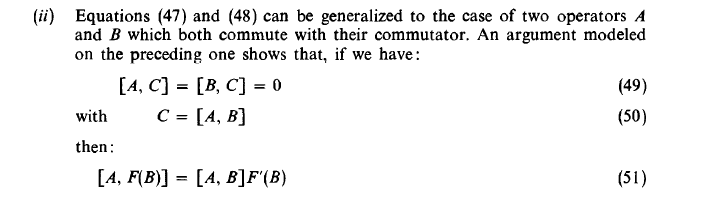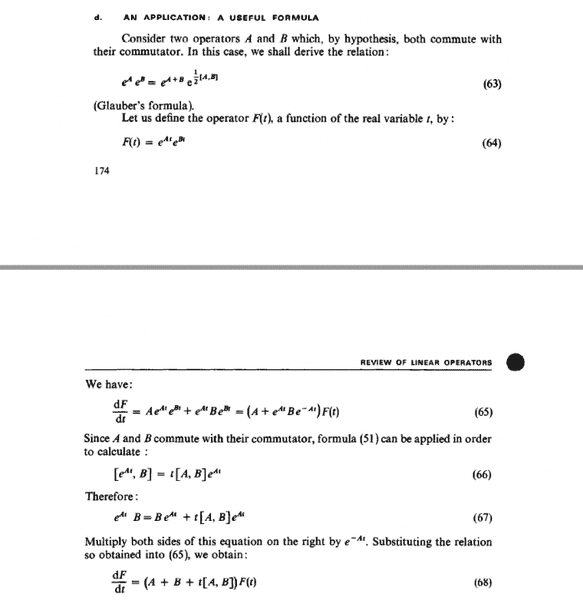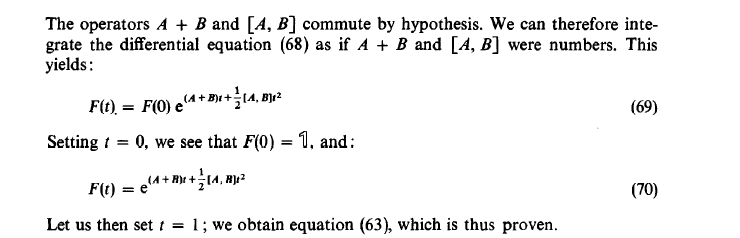# About Glauber's formula(or special Baker–Campbell–Hausdorff

• A
• Frost_Xue

#### Frost_XueHi, I don't understand how to use 51 get 66. Can you help me with that?

Make the following replacement: ##F(B) = e^{At}## and ##A=B##.

Make the following replacement: ##F(B) = e^{At}## and ##A=B##.
ok if I replace F(B) = e^At then should it be [A,B](e^At)' ? how does the t come from? and what do you mean by A=B? also the order of commutator seems reversed shouldn't it be negative then?
Sorry I am stupid.

##(e^{At})' = \frac{d}{dA}e^{At}##.

Yes, the order is reversed on both sides of the equation. So there will be negative sign in each side and they can cancel out.

##(e^{At})' = \frac{d}{dA}e^{At}##.

Yes, the order is reversed on both sides of the equation. So there will be negative sign in each side and they can cancel out.
oh I didn't pay attention on the order of the commutator on the right hand side. Thank you so much for the help and time. And sorry for bothering you again.

No worry, it's not a big deal.

Which book is this from?

C. Cohen-Tannoudji et al. - Quantum Mechanics.

•Demystifier